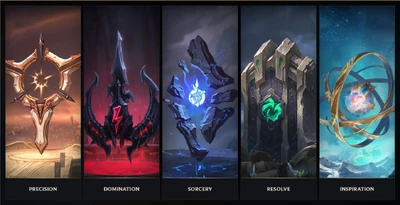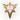1,825 個頁面## 符文系

•精密（強化攻擊和持續傷害）
•主宰（爆發傷害並前往目標）
•巫術（強化技能和資源控制）
•堅決（耐久和控制）
•啟迪（創造性的工具並扭轉常規）### 屬性碎片

Option 1 Option 2 Option 3
Slot 1
Offense5.4 bonus Attack Damage or 9 Ability Power (Adaptive)10% bonus attack speed1% − 10% (based on level) cooldown reduction
Slot 2
Flex5.4 bonus Attack Damage or 9 Ability Power (Adaptive)6 bonus armor8 bonus magic resistance
Slot 3
Defense15 − 90 (based on level) bonus health6 bonus armor8 bonus magic resistance

### 轉換

When the game starts the chat informs of these substitutions.

## 符文頁Rune page inventory

• 1-9級的玩家無法新建或編輯符文頁。
• 10級開始可以新建和編輯符文頁。
• 每個新帳號有3個空白符文頁。可以在商城花費或購買額外的符文頁。
• S8季前賽階段符文系統重鑄，玩家在重鑄前擁有的符文頁會轉為新的符文頁，並會收到額外的補償。
• 每個帳號最多有25個符文頁（不包括預設符文頁）。
• 你可以選擇隱藏預設符文頁。

## Trivia

• During development, Precision and Sorcery were originally going to be Primary path only.
• Runes and their surrounding areas have distinct visual appearances which bear similarities to certain Runeterran factions. This may be intentional in order to reflect the location of the World Runes:
•Precision - Golden cityscape of
•Domination - fortification
•Sorcery - Mountains of
•Resolve - Riverlands of Southern
•Inspiration - Beneath the heavens atop
• The following statements generalize the changes made to compensate the loss of AD from Marks and Health/Armor from Seals, which were almost unanimously used due to the lack of options (and thus the game has been balanced, for example, around most champions having +9 Armor from Seals). The exact values may vary for specific cases, as Riot also took this opportunity to better distinguish classes (e.g. tanks from non-tanks; ranged from melee), as well as buff/nerf specific champions.
• AD champions had the base damage on their abilities increased by 5.
• Ranged AD champions also had their increased by 8.
• Most champions had their increased by 9.
• Most mages had their increased by 12 (+12), instead. Primarily excepting junglers.
• Melee supports had their increased by 20, instead.
• Every rune that gives bonus Adaptive AD or AP, will always grant about 60% of the amount of AP as AD, or about 166.6% of the amount of AD as AP.
• More runes and paths may be added in the game.
• In Year in Review 2017 a joke phrase was added to each champion. A list with all phrases can be found over here.
• The number of all rune combinations can be seen below.
Calculation of rune combinations
Count Paths Calculation
Primary path Secondary path Primary path Secondary path Total Combinations
1PrecisionDomination 4*3*3*3=108 3*3+3*4+4*3=33 108*33=3564
1PrecisionSorcery 4*3*3*3=108 3*3+3*3+3*3=27 108*27=2916
1PrecisionResolve 4*3*3*3=108 3*3+3*3+3*3=27 108*27=2916
1PrecisionInspiration 4*3*3*3=108 3*3+3*3+3*3=27 108*27=2916
1DominationSorcery 4*3*3*4=144 3*3+3*3+3*3=27 144*27=3888
1DominationResolve 4*3*3*4=144 3*3+3*3+3*3=27 144*27=3888
1DominationInspiration 4*3*3*4=144 3*3+3*3+3*3=27 144*27=3888
1SorceryResolve 3*3*3*3=81 3*3+3*3+3*3=27 81*27=2187
1SorceryInspiration 3*3*3*3=81 3*3+3*3+3*3=27 81*27=2187
1ResolveInspiration 3*3*3*3=81 3*3+3*3+3*3=27 81*27=2187
1DominationPrecision 4*3*3*4=144 3*3+3*3+3*3=27 144*27=3888
1SorceryPrecision 3*3*3*3=81 3*3+3*3+3*3=27 81*27=2187
1ResolvePrecision 3*3*3*3=81 3*3+3*3+3*3=27 81*27=2187
1InspirationPrecision 3*3*3*3=81 3*3+3*3+3*3=27 81*27=2187
1SorceryDomination 3*3*3*3=81 3*3+3*4+4*3=33 81*33=2673
1ResolveDomination 3*3*3*3=81 3*3+3*4+4*3=33 81*33=2673
1InspirationDomination 3*3*3*3=81 3*3+3*4+4*3=33 81*33=2673
1ResolveSorcery 3*3*3*3=81 3*3+3*3+3*3=27 81*27=2187
1InspirationSorcery 3*3*3*3=81 3*3+3*3+3*3=27 81*27=2187
1InspirationResolve 3*3*3*3=81 3*3+3*3+3*3=27 81*27=2187
Total

• Prior to the introduction of Shards, players received a bonus based on the combination of their sets (e.g. Sorcery + Precision). These combinations had specific Titles, which are shared with the corresponding presets. The following is listed for archive purposes:
Secondary ► Precision Domination Sorcery Resolve Inspiration
Primary ▼
Precision The Merciless Elite The Brazen Prefect The Eternal Champion The Savant
Domination The Twisted Surgeon The Aether Blade The Immortal Butcher The Wicked Maestro
Sorcery The Incontestable Spellslinger The Calamity The Ancient One The Cryptic
Resolve The Imperious Behemoth The Leviathan The Arcane Colossus The Enlightened Titan
Inspiration The Elegant Duelist The Ruthless Visionary The Stargazer The Timeless
• 'The Perfect' preset was originally called 'The Prefect', as with the Set Title. It is unknown why this was changed.